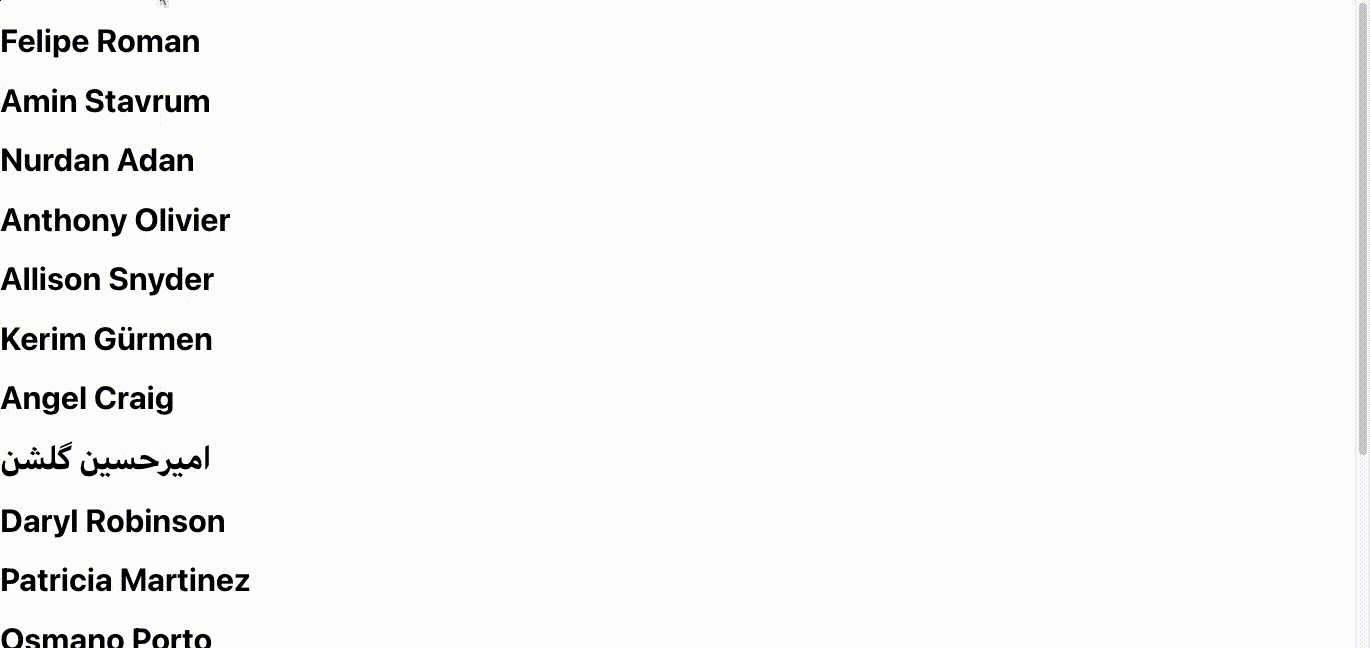# .css-4zleql{display:block;}Rahul Banerjee# How to use APIs with React Functional Components

Subscribe to my newsletter and never miss my upcoming articles

Using APIs in your react project is a common use case. In this tutorial, we will be looking at two use cases

We will be using functional components and the useEffect hook. Some familiarity is expected.In this use case, the data is only loaded once - whenever the user views the app or refreshes the page. Initially, a 'Loading…' text is shown. This text is later updated with the actual API data. Below is the code snippet of the component which causes the above behavior

Let's discuss the code in 3 parts, the states, the useEffect hooks, and the rendering logic

### The states

``````const [isLoading, setIsLoading] =
React.useState(true);``````

We have two states. The isLoading state is a boolean variable initialized to True. This state is used to keep a track of whether the data is still loading or it has already been loaded. The setIsLoading function is used to toggle this state variable. After the API returns the data, we will use this function to toggle the value for isLoading

``const [data, setData] = React.useState([]);``

Next, we have the data state. This state is initialized to an empty array. It will be used to store the data returned by the API. You can initialize the state to an empty object as well. However, the API I am using in the example returns a list and therefore an empty list seems like the right choice. The setData function is used to update the state variable data after the API returns the data.

### The useEffect Hooks

``````  React.useEffect(() => {
const url = "https://randomuser.me/api/?results=15";
fetch(url)
.then((response) => response.json())
.then((json) => setData(json['results']))
.catch((error) => console.log(error));
}, []);``````

The above useEffect Hook is used to make the request to the API. The '[]' parameter tells React to run this hook only once. The hook runs after the page has loaded. A simple fetch request is made and after the promise(s) are resolved, we use the setData function to update the state variable data

``````  React.useEffect(() => {
if (data.length !== 0) {
}
console.log(data);
}, [data]);``````

The next useEffect hook runs whenever the state variable data is updated. It does a simple check, if the state variable data is not empty, i.e the API has returned the data, it sets the state variable isLoading to False.

Note: The above case assumes a happy case, i.e the API will always return the data. However, this is not true. Therefore error handling should also be added.

### The rendering logic

``````  return (
<div>
) : (
data.map((user) => (
<h1>
{user.name.first} {user.name.last}
</h1>
))
)}
</div>
);
}``````

The rendering logic is pretty straightforward, if the state variable 'isLoading' is True, we will display the 'Loading…' indication. If it is false, we simply map over the state variable 'data' and display all the items in the array.Below is the entire code snippet

We will discuss the code in 3 parts.

### States

`````` const [showData, setShowData] =
React.useState(false);``````

The first two state variables are the same as the ones in the previous section. We will discuss the third state variable showData.

When the user views the page for the first time, we do not want them to see the API data or the 'Loading……' text. Therefore we add a simple check to see if the user has clicked the button. After the user clicks the button once, there are only two views

• The API Data

Every time the user clicks the button again, we just toggle between the two views mentioned above.

### Handle Click Function

``````  const handleClick = () => {
setShowData(true)
const url = "https://randomuser.me/api/?results=15";
fetch(url)
.then((response) => response.json())
.then((json) => {
setData(json["results"])
console.log(data);
})
.catch((error) => console.log(error));
};``````

This is similar to the first useEffect Hook in the first use case. The only difference is that we set our state variable showData to True.

### Rendering logic

``````return (
<div>
{showData ? (
) : (
data.map((user) => (
<h1>
{user.name.first} {user.name.last}
</h1>
))
)
) : (
<div></div>
)}
</div>
);``````

First, we have a check for showData, this is to ensure that initially, the user doesn't see the 'Loading….' text nor the API data. After the user clicks the button, showData is set to True. After this, the rendering logic is similar to the first use case.